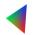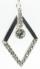# Area + expression of a variable from the formula - math problems

#### Number of problems found: 442

• 7 triangleThe triangle area is 26.7 cm2. Determine the side length l if appropriate height hl = 45.3 cm.
• TerezaThe cube has an area of base 256 mm2. Calculate the edge length, volume, and area of its surface.
• CylinderThe cylinder surface is 922 dm2. Its height is equal to the radius of the base. Calculate the height of this cylinder.
• R TrapeziumRectangular trapezium has bases 21 and 10 and area 92 cm2. What is its perimeter?
• Rectangular cuboidThe rectangular cuboid has a surface area 5334 cm2, and its dimensions are in the ratio 2:4:5. Find the volume of this rectangular cuboid.
• ConeCircular cone of height 15 cm and volume 5699 cm3 is at one-third of the height (measured from the bottom) cut by a plane parallel to the base. Calculate the radius and circumference of the circular cut.
• Right ΔA right triangle has the length of one leg 11 cm and the hypotenuse 61 cm size. Calculate the height of the triangle.Determine the radius of the circle if its perimeter and area is the same number.
• Cone A2VThe surface of the cone in the plane is a circular arc with central angle of 126° and area 415 cm2. Calculate the volume of a cone.
• Right isoscelesCalculate area of the isosceles right triangle which perimeter is 26 cm.
• PipeHow long is the pipe with an outside diameter of 0.87 m if his coloring consumed 10 kg of color. 1 kg of color coverage is 6 m2.
• Arc and segmentCalculate the length of circular arc l, area of the circular arc S1 and area of circular segment S2. Radius of the circle is 11 and corresponding angle is (2)/(12) π.
• Heron backlawCalculate missing side in a triangle with sides 25 and 13 and area 152.
• CircleHow big is an area of a circle if its circumference is 51.2 cm?
• CapJesters hat is shaped by a rotating cone. Calculate how much paper is needed to the cap 54 cm high when the head circumference is 47 cm.
• Right triangleLegs of the right triangle are in the ratio a:b = 2:8. The hypotenuse has a length of 87 cm. Calculate the perimeter and area of the triangle.
• Sphere A2VThe surface of the sphere is 241 mm2. What is its volume?
• GardenTrapezoid garden has parallel sides 19 m and 24 m. Its area is 193.5 square meters. What is the width of the garden?
• ShapePlane shape has a maximum area 677 mm2. Calculate its perimeter if perimeter is the smallest possible.
• Rhombus problemThe perimeter of the rhombus is 268 m, and its area is 2278 m2. Calculate the height of the rhombus.

Do you have an exciting math question or word problem that you can't solve? Ask a question or post a math problem, and we can try to solve it.

We will send a solution to your e-mail address. Solved examples are also published here. Please enter the e-mail correctly and check whether you don't have a full mailbox.

Area - math word problems. Expression of a variable from the formula - math word problems.